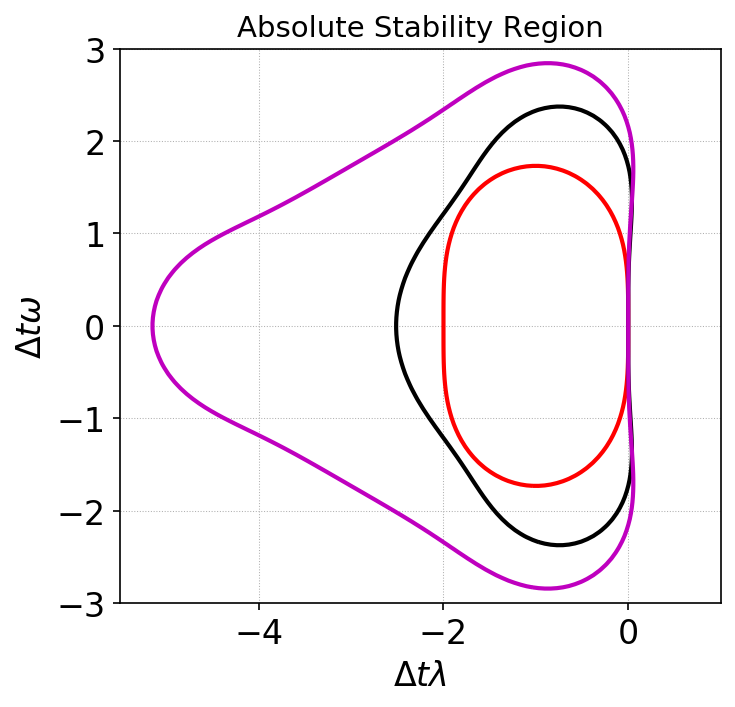# Strong-Stability preserving Runge-Kutta time-steppers¶

The Gkyl DG solvers use SSP-RK time-steppers. Three steppers are implemented: SSP-RK2, SSP-RK3 and a four-stage SSP-RK3 that allows twice the CFL (for the cost of additional memory) as the other schemes. See [DurranBook] page 56. The schemes are described below. Here, the symbol $$\mathcal{F}$$ is used to indicate a first-order Euler update:

$\mathcal{F}[f,t] = f + \Delta t \mathcal{L}[f,t]$

where $$\mathcal{L}[f]$$ is the RHS operator from the spatial discretization of the DG scheme.

## SSP-RK2¶

$\begin{split}f^{(1)} &= \mathcal{F}[f^{n},t^n] \\ f^{n+1} &= \frac{1}{2} f^{n} + \frac{1}{2}\mathcal{F}[f^{(1)},t^n+\Delta t]\end{split}$

with $$CFL \le 1$$.

## SSP-RK3¶

$\begin{split}f^{(1)} &= \mathcal{F}[f^{n},t^n] \\ f^{(2)} &= \frac{3}{4} f^{n} + \frac{1}{4}\mathcal{F}[f^{(1)},t^n+\Delta t ] \\ f^{n+1} &= \frac{1}{3} f^{n} + \frac{2}{3}\mathcal{F}[f^{(2)},t^n+\Delta t/2]\end{split}$

with $$CFL \le 1$$. As this scheme has three stages instead of two, it will take about $$1.5X$$ longer to run than the SSP-RK2 scheme.

## Four stage SSP-RK3¶

$\begin{split}f^{(1)} &= \frac{1}{2} f^{n} + \frac{1}{2} \mathcal{F}[f^{n},t^n] \\ f^{(2)} &= \frac{1}{2} f^{(1)} + \frac{1}{2} \mathcal{F}[f^{(1)},t^n+\Delta t/2] \\ f^{(3)} &= \frac{2}{3} f^{n} + \frac{1}{6} f^{(2)} + \frac{1}{6} \mathcal{F}[f^{(2)},t^n+\Delta t] \\ f^{n+1} &= \frac{1}{2} f^{(3)} + \frac{1}{2} \mathcal{F}[f^{(3)},t^n+\Delta t/2]\end{split}$

with $$CFL\le 2$$. Note that this scheme has four stages, but allows twice the time-step that SSP-RK2 and SSP-RK3, hence will result in a speed up of $$1.5X$$ compared to the three-stage SSP-RK3 scheme.

## Region of absolute stability¶

For each of the above schemes, I have plotted below the region of absolute stability. Note that only the RK3 schemes are stable when there is no diffusion in the system, and hence should be prefered.Absolute stability regions for a equation $$\dot{y} = (\lambda+i\omega)y$$ for SSP-RK2 (red), SSP-RK3 (black) and four stage SSP-RK3 (magenta). When there is no diffusion ($$\lambda=0$$) the SSP-RK2 scheme is slightly unstable as it has no intercept on the imaginary axis. Hence, the third order schemes should be preferred.